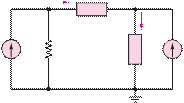### Create an Account

Home / Questions / The equivalent circuit of Figure P373 has VT 12 V RT 8 Q If the conditions fo...

# The equivalent circuit of Figure P373 has VT 12 V RT 8 Q If the conditions for maximum power transfer exist Figure P370 determine a The value of RL b The power

The equivalent circuit of Figure P3.73 has

VT  = 12 V        RT  = 8 Q

If the conditions for maximum power transfer exist,

Figure P3.70

determine

a.    The value of RL.

b.    The power developed in RL.

Do not solve the resulting equations.

c.    The efficiency of the circuit, that is, the ratio of power absorbed by the load to power supplied by the source.

iav

1

1 A                  1 Ù

Ra

 v

2

+  va   –            ib

+

Rb          vb

Jun 19 2020 View more View LessSubscribe To Get Solution